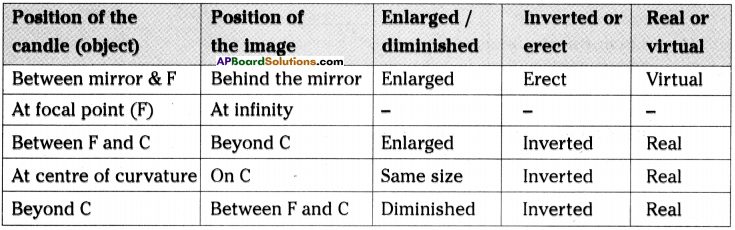# AP Board 9th Class Physical Science Solutions Chapter 7 Reflection of Light at Curved Surfaces

AP State Syllabus AP Board 9th Class Physical Science Solutions Chapter 7 Reflection of Light at Curved Surfaces Textbook Questions and Answers.

## AP State Syllabus 9th Class Physical Science Solutions 7th Lesson Reflection of Light at Curved Surfaces

### 9th Class Physical Science 7th Lesson Reflection of Light at Curved Surfaces Textbook Questions and Answers

Question 1.
“Laws of reflection of light are not applicable to curved surfaces” Is it correct?
No, laws of reflection of light are applicable to curved surfaces also.

Question 2.
How do you find the focal length of a concave mirror?
(OR)
What is the name given to distance between pole and focal point? How would you able to find that distance by using an activity? That distance is called focal length.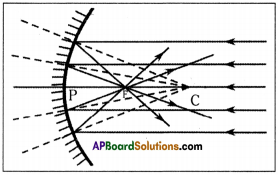To find the focal length of a concave mirror –

1. Hold a concave mirror perpendicular to the direction of sunlight.
Take a small paper and slowly move it in front of the mirror and find out the point where you get smallest and brightest spot, which is image of the sun.
The rays coming from sun parallel to the concave mirror are converging at a point.
This point is called Focus or focal point (F) of the concave mirror.
Measure the distance of the spot from the vertex (point P) of the mirror.
This distance gives the focal length of the concave mirror.

Question 3.
Where will the image form when we place an object, on the principal axis of a concave mirror at a point between focus and centre of curvature?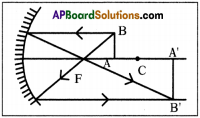• When we place an object on the principal axis of a concave mirror at a point between focus and centre of curvature then the image will form at a point beyond the centre of curvature.
• The image is enlarged, inverted and real.Question 4.
Find the distance of the image, when an object is placed on the principal axis at a distance of 10 cm in front of a concave mirror whose radius of curvature is 8 cm.
(OR)
If the object is placed on the principal axis at a distance of 10 cm in front of a concave mirror with curvature is 8 cm, what is the distance of the image?
Object distance u = – 10 cm
Radius of curvature (R) = – 8 cm
∴ Focal length f = $$\frac{R}{2}=\frac{-8}{2}$$ = – 4 cm
Image distance = v = ?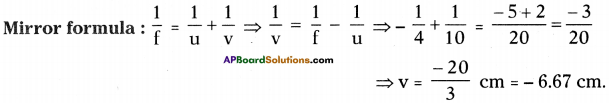∴ The image distance = 6.67 cm.
i.e., Real image is formed at same side of the mirror.

Question 5.
State the differences between convex and concave mirrors.
(OR)
Distinguish between convex and concave mirrors.
(OR)
Ramu observed a mirror which is used by drivers to see the vehicles behind. Srinu observed a mirror which is used by dentist to see teeth inside the mouth. What are those two mirrors and distinguish them?
Those mirrors are (1) Convex, (2) Concave

 Convex mirror Concave mirror 1. After reflection from the mirror the light rays diverge. 1. After reflection from the mirror the light rays converge. 2. The reflecting surface curved outward. 2. The reflecting surface curved inward. 3. Radius of curvature and focal length are taken as positive, in sign conversion. 3. Radius of curvature and focal length are taken as negative, in sign conversion. 4. It’s magnification is positive only. 4. It’s magnification may be both positive and negative. 5. Magnification of convex mirror is in between zero and one. 5. Magnification value of concave mirror having all values. 6. The image formed due to convex mirror is always virtual. 6. The image formed due to concave mirror is generally real. But when an object placed between vertex and focus it is virtual. 7. The image formed due to convex mirror is always diminished. 7. The image formed due to concave mirror is diminished or magnified or same size based on position of object. 8. The image due to convex mirror is always erect. 8. Generally the image is inverted except when the object is placed between P and F.

Question 6.
Distinguish between real and virtual images.
(OR)
Varun sees his image in a concave mirror. When he moves away he was unable to see his image. Write the differences between those two images.

 Real image Virtual image 1. Real image is formed due to converging of light rays. 1. Virtual image is formed due to diverging of light rays. 2. This image can be formed on screen. 2. This image cannot be formed on screen. 3. Generally this image is inverted. 3. Generally this image is erect. 4. It cannot be seen in the mirror. 4. It can be seen in the mirror.

Question 7.
How do you get a virtual image using a concave mirror?
(OR)
Ramu moved an object towards a concave mirror. When he reached certain point he was able to see his image. Where does he had placed the object inorder to see the object in the mirror?

• Place the object at a distance less than the focal length of the mirror.
• Draw ray diagram as shown in the figure.
• When the rays are extended, they seem to be coming out from a point on the other side of the mirror. (G)
• We cannot find the image on the screen, but we can see the image in the mirror. (IG)
• This is the virtual, erect and magnified image.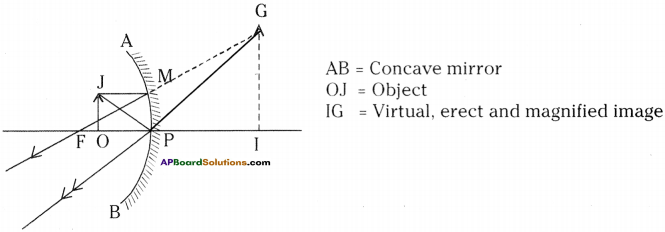Question 8.
What do you know about the terms given below related to the spherical mirrors?
a) Pole
b) Centre of curvature
c) Focus
e) Focal length
f) Principal axis
g) Object distance
h) Image distance
i) Magnification
a) Pole :
The centre of the spherical mirror is called pole of the mirror.

b) Centre of curvature :
The normal from a concave mirror converge at a point, that point is called centre of curvature.

c) Focus :
A point on the principal axis where a beam of light parallel to the principal axis either converges into or appears to diverge from after reflection from the mirror is called focus (or) focal point (F).

The distance between pole and centre of curvature is called radius of curvature. It is denoted by ‘R’.
R = 2f

e) Focal length :
The distance of the focus from the pole is called the focal length (f) of the mirror.

f) Principal axis :
The horizontal line which passes through the centre of curvature and pole.

g) Object distance :
The distance between object and mirror is called object distance (u).

h) Image distance :
The distance between image and mirror is called image distance (v).

i) Magnification :
The ratio of size of image to the size of object is known as magnification.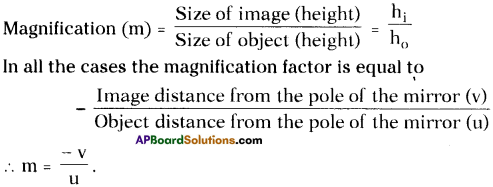Question 9.
Write the rules for sign convention.
(OR)
Vinay wants to solve a problem related to mirrors. What are rules to be followed by him in order to solve the problem?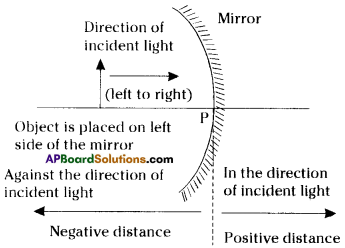Sign conventions used in mirror equation :

1. All distances should be measured from the pole.
2. All distances measured in the direction of incident ray are to be taken as positive.
3. The distances measured in the direction opposite to incident ray are to be taken as negative.
4. Height of object (h0) and height of image (hi) are positive, if measured above principal axis and negative if measured below principal axis.

Question 10.
The magnification produced by a convex mirror is – 1. Do you agree it?
The magnification produced by a convex mirror is – 1.1 agree with this statement.Question 11.
Imagine that spherical mirrors were not known to human beings. Guess the consequences.
(OR)
What are the consequences occur when the spherical mirrors were not known to human beings?

• Spherical mirrors are used by human beings in their daily life.
• Convex mirrors are used in hospitals, hotels, stores, apartments, roads, ATMs, computer monitors, rare-view mirrors in vehicles, and also in sun glasses,
• Whereas concave mirrors are used by ENT specialists, dentists to see inner parts of mouth,
• Car head lights are used to get parallel beam of light,
• Solar cookers are also made from concave mirrors, So everywhere we are using these spherical mirrors,
• So without these spherical mirrors the life of human beings is miserable,

Question 12.
By observing steel vessels and different images in them, Surya, a third class student, asked his elder sister Vidya some questions. What may be those questions?
(OR)
By observing the image on steel vessels, Surya asked his sister some questions. What may be those questions?

• Why do we see our image in some vessels which bulged outwards?
• Why are we not able to see our image in some vessels which bulged inwards?
• Which type of vessels are useful to reflect light on wall and form our image on wall?
• Can we see the images in all vessels?
• Is these images real or virtual?
• The vessels which are bulged inside behaves like which type of mirrors?Question 13.
How do you form a diminished image by a concave mirror on a screen?
If we place an object beyond centre of curvature of a concave mirror, diminished image will be formed between focus and centre of curvature,

Question 14.
How do you find the focal length of a concave mirror in the lab?
Aim :
To find out focal length of a concave mirror,

Material required :
A candle, paper, concave mirror, V-stand, measuring tape, or meter scale.

Procedure:
1) Place the concave mirror on V-stand, a candle, and meter scale as shown in figure.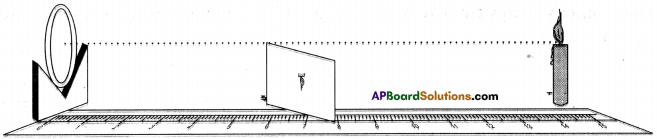2) Keep the candle at different distances from the mirror (10 cm to 80 cm) along the axis and by moving the paper (screen) find the position where you get the sharp image on paper.

3) Note the distance of candle from the mirror (u) and distance of the image from the mirror (v) in the given table.
Here u = object distance, v = image distance, f = focal length.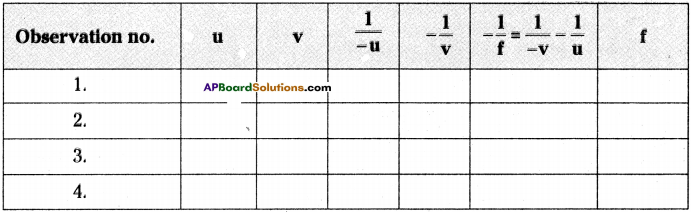4) Find the average of T. This value of T is the focal length of the given concave mirror.Question 15.
What do you infer from the experiment which you did with concave mirrors and measured the distance of object and distance of image?
I observed the following points from the experiment with concave mirrors, to form images at different places, they are :

1. As the object distance increases, the image distance decreases.
2. As the object distance increases, the size of image decreases.

Question 16.
Draw a ray diagram to show the process of converging of the parallel beam by a concave mirror by taking four parallel incident rays.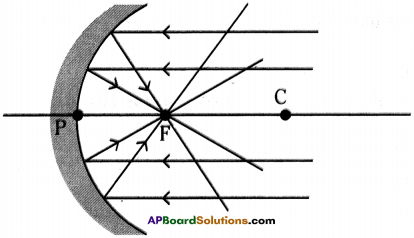Question 17.
Draw suitable rays by which we can guess the position of the image formed by a concave mirror.
Suitable rays by which we can guess the position of the image formed by a concave mirror.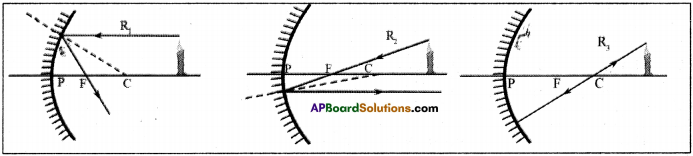Rule 1 :
All the rays, that are parallel to the axis, get reflected such that they pass through the focal point of the mirror.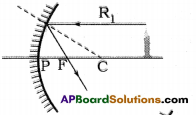Rule 2 :
A ray that passes through the focal point of the mirror will, travel parallel to the axis after reflection.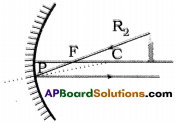Rule 3 :
A ray, passing through the centre of curvature of the mirror and incidents on the mirror, after reflection will travel along the same line, but in opposition direction.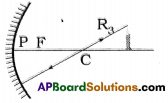Question 18.
Show the formation of image with a ray diagram, when an object is placed on the •principal axis of a concave mirror away from the centre of curvature.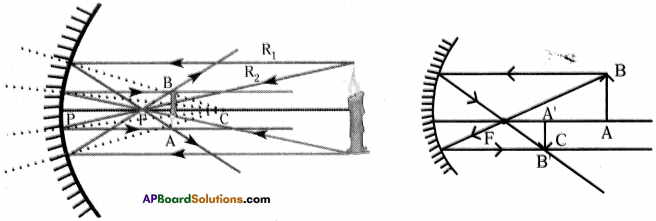Question 19.
Make a solar heater / cooker and explain the process of making.
(OR)
Which cooker uses solar energy to cook food and explain its making?
(OR)
Explain the construction and working of a solar cooker.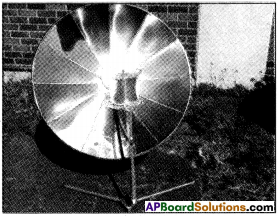Construction:

1. Make a wooden / iron frame in dish shape. It is solar cooker.
2. Cut the acrylic mirror sheets into 8 or 12 pieces in the shape of isosceles triangles with a height equal to the radius of a dish antenna.
3. The bases of 8 or 12 triangles together make the circumference of the dish.
4. Stick the triangle mirrors to the dish as shown in figure.
5. Thus solar heater/cooker is ready.

Working:

1. Face it towards the sun.
2. Find its focal point and place a vessel at that point.
3. It will get heated. Everyone cook rice in that vessel.Question 20.
To form the image on the object itself, how should we place the object in front of a concave mirror? Explain with a ray diagram.
(OR)
Draw ray diagram to explain the image formation on the concave mirror if the object is in front of it.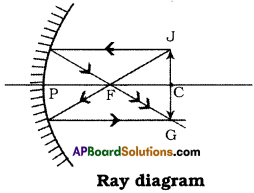• The object should be placed on centre of curvature of concave mirror.
• When the object placed at centre of curva¬ture after reflection from the mirror the light rays converge at centre of curvature.
• So a real and inverted image is formed on the object itself. Ray diagram

Question 21.
How do you appreciate the role of spherical mirrors in our daily life?
(OR)
Write the usage of spherical mirror in daily life situations.

• Spherical mirrors are useful in our daily life in many ways.
• Convex mirrors are used as rear view mirrors in cars, scooters, buses, etc. This helps us to see the traffic behind the vehicle, which avoids accidents while taking turns.
• Big convex mirrors are used as shop security mirrors.
• Concave mirrors are used by dentists, opthamologists, to see the smaller parts of teeth, eyes, and ears.
• Concave mirrors are also used in solar heating devices.
• Concave mirrors are used as shaving mirrors to see a large image of the chin (or) face.
• Concave mirrors are used as doctor’s head mirrors to focus light coming from a lamp on to the body parts of the patient to be examined by the doctor.
• So, I appreciate the role of spherical mirrors in our daily life.Question 22.
How do doctors use concave mirrors?

• Dentists and ENT specialist doctors use concave mirrors to get a magnified clearer view of the teeth, skin and ear cavities.
• A concave mirror converges the rays of light falling on it in a direction parallel to the principal axis, on to its focus. This is the reason why ENT specialists use it as a head mirror.

Question 23.
Why do we prefer a convex mirror as a rear-view mirror in the vehicles?

• Convex mirror always gives an erect and diminished image.
• The image distance will be smaller than the object distance.
• A convex mirror has a wider field of view than a plane mirror.
• Hence we prefer convex mirror as rear-view mirror in the vehicles, as we have to observe the vehicles which are at the back of our vehicle.

Question 24.
Complete the table-1 which is related to experiment done by a concave mirror.

 Object distance Image distance Magnification 1. 10 cm 2. 20 cm 3. 30 cm 4. 40 cm 1

 Object distance Image distance Magnification 1. 10 cm 20 cm 2 2. 20 cm Infinity Cannot measure 3. 30 cm 60 cm 2 4. 40 cm 40 cm 1

From the given data, m = $$\frac{40 \mathrm{~cm}}{40 \mathrm{~cm}}$$ cm = 1.
Hence, R = 40 cm, f = 20 cm.Question 25.
How can you show the diverging and the converging of light by using laser lights?
Aim :
To show diverging and converging of light by using laser lights.

Material required :
Concave mirror, convex mirror, laser lights-2, screen, V-stand, Agarbathi.

Procedure:

• Place a concave mirror on a V-stand and place the V-stand on a table.
• Take two laser lights.
• Focus the light rays of laser lights parallel to the axis of the concave mirror.
• The light rays (beams) incident on the concave mirror are reflected back and converge at one point.
• Place the screen to catch that converging point of reflected light rays.
• Light a Agarbathi near the table.

Observation :
We can observe path of the incident and reflected rays clearly in the smoke of Agarbathi.

• Now place a convex mirror on the stand.
• Again pass the laser light rays parallel to the principal axis at the convex mirror.

Observation :
We can observe diverging light rays. We cannot catch any converging point on the screen.

Question 26.
Collect information about the history of spherical mirrors in human civilization. Display it in your classroom.

• The idea of mirror came into existence long back when people saw their images in water, on poluted surfaces, etc.
• The history of mirrors starts in the 6000 BC. The earliest man made mirrors were pieces of poluted stone.
• The first glass mirrors were produced in 1A.D. by Romans.
• The first modern silver-glass mirror was created by Justus Von Liebig, a German chemist in 1835.
• The invention of glass blowing method during the 14th century led to the discovery of spherical mirrors, which increased the popularity of glass mirrors.
• By the end of 18th century, decorative mirrors have widely used.
• New, cheaper techniques of mirror production in the 19th century led to a great proliferation in their use.

Question 3.
Think about the objects which act as a concave or convex mirrors in your surroundings. Make a table and display it in your classroom.
(OR)
Collect some objects from your surroundings that act as concave, convex mirrors and display them your classroom.
Object used as convex and concave mirror in our surroundings.

 Convex mirror Concave mirror 1. Spoon bulged outwards. 1. Spoon bulged inwards. 2. Outer surface of cooking vessel. 2. Inner surface of cooking vessel. 3. Sink outwards. 3. Sink (wash basin) inwards. 4. Glass outwards. 4. Glass inwards.

Question 4.
How will our image be in concave and convex mirrors? Collect photographs and display in your classroom.

• In concave mirrors our image is thin and enlarged.
• As we move away from mirror the image will be diminished and become pointed at the pole.
• In convex mirror, our image is bulged and size of image is diminished.
• As we move away from the mirror, the image is further diminished.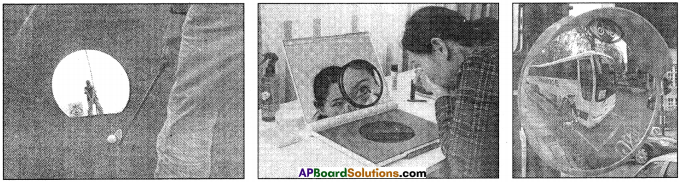Question 5.
How do you appreciate the use of reflection of light by a concave mirror in making of TV antenna dishes?

• The parabolic shape of dish reflects the signals to the dish’s focal point.
• Mounted on brackets at the dish’s focal point is a device called a feed horn.
• This feed horn is a wave guide that gathers the signals at or near the focal point and conducts them to a LNB (Low-Noise Block down converter).
• The LNB converts these electromagnetic waves into electrical signals and shifts to the receiver i.e. T.V. set.
• This is all possible only with the help of parabolic dish antennas (concave shape).
• We know, the T.V. plays an important role in our daily life. So the inventions of the utilities of concave shapes are more helpful for us.Question 6.
A convex mirror with a radius of curvature of 3 m is used as rear view in an automobile. If a bus is located at 5 m from this mirror, And the position, nature and size of the image.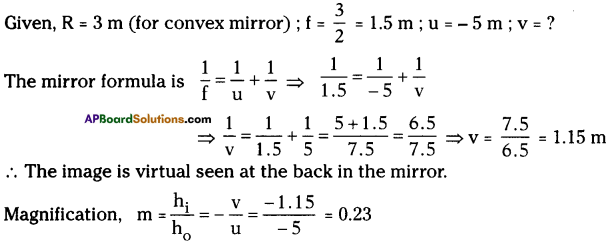Position = 1.15 m behind the mirror. Nature = Virtual and erected.
∴ Image is erect and smaller in size by a factor of 0.23.

Question 7.
An object is placed at a distance of 10 cm from a convex mirror of focal length 15 cm. Find the position and nature of the image.
(OR)
An object 5 cm high is placed at a distance of 10 cm from a convex mirror, the focal length of the mirror is 15 cm. Find the nature, position and size of the image.
(v = +6m ; m = 0.6, h2 = 3 cm)
Given, u = – 10 cm ; f = 15 cm ; v =?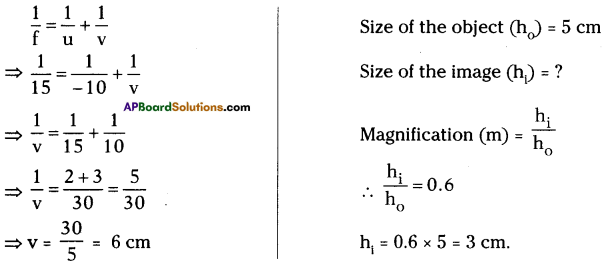So the Image is virtual and seen in the mirror.
Magnification m = $$-\frac{v}{u}=\frac{-6}{-10}=\frac{6}{10}$$ = 0.6
Position : 6 cm behind the mirror.
Nature : Virtual and erect image.
So the image is erect and diminished.

Question 8.
Write answers to the following questions based on the data given in the table – 3.
1) What changes will come gradually in the size of an image, when we move an object away from the concave mirror?
2) In which situation inverted images formed by a concave mirror?
3) If the centre of curvature of a concave mirror is 10 cm, where the object should be placed to get an image at centere of curvature?
1) a) If we move an object from a concave mirror to its focus (F), we will get a virtual image.
b) If we place the object at ‘F, the size of the image is infinity.
c) If we move, the object from ‘F to centre of curvature the size of the image is decreases gradually but the size of the image is bigger than that of the size of the object.
d) At ‘C the size of the image is same as the size of the object.
e) From ‘C’ to infinity distance the size of the image is smaller than the siz4 of\$he object and decreases gradually.
f) Hence, the size of the image decreases if we move an object away from the focus fo the concave mirror.

2) If we place an object beyond focus (F) inverted image will be formed.

3) At 10 cm distance (or) at u = 10 cm.

### 9th Class Physical Science 7th Lesson Reflection of Light at Curved Surfaces InText Questions and Answers

9th Class Physical Science Textbook Page No. 111

Question 1.
Did you burn a paper by using a magnifier?
Yes.

Question 2.
What causes burning of the paper, while doing it?
Converging light rays through magnifier at one point on the paper.

Question 3.
Can you use a plane mirror to burn a paper instead of a magnifier lens?
Cannot.Question 4.
Can’t? Why?
Plane mirror cannot converge light rays.

Question 5.
Which type of mirrors may be used to converge light rays?
Concave mirrors.

Question 6.
Which type of reflected surface used by Archimedes?
Curved reflected surfaces.

9th Class Physical Science Textbook Page No. 112

Question 7.
Can we burn a paper by using light rays of the torch light?
Cannot.

Question 8.
Can’t? Why?
The light rays coming from the torch light are not parallel rays. Hence, they cannot converge at one point on the paper with high intensity.Question 9.
Which type of incident rays are required to get high intensity and point sized converging point?
Parallel light rays.

Question 10.
Which line is taken as a base to draw a reflected ray to the given incident ray?
Normal taken as base.

9th Class Physical Science Textbook Page No. 114

Question 11.
Does a concave mirror from image at focus in all situations?
No

9th Class Physical Science Textbook Page No. 114

Question 12.
See the given figure, a set of parallel rays are falling on a convex mirror. What conclusions can you draw from this?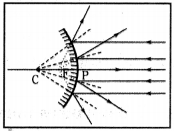The parallel rays are diverging after reflection. If we extend the reflected rays backwards, they meet at F which is focal point of convex mirror. The image formed is virtual, straight and point sized.Question 13.
Will you get a point image if you place a paper at the focal point?
We can get highly enlarged image at infinity when we place paper at focal point.

9th Class Physical Science Textbook Page No. 118

Question 14.
How can you find the focal length of a convex mirror?

• The focal length of a convex mirror can be determined by introducing a convex lens between the object and the convex mirror.
• An image can be obtained with the help of a convex lens side by side with object when the convex mirror reflects the rays along the same path.
• Then, the radius of curvature (R) of the mirror is the distance between the screen and the mirror.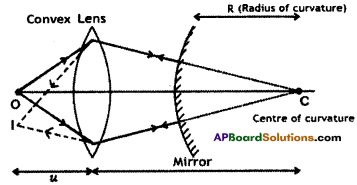• The focal length ‘f of the convex mirror is calculated using the formula R (Radius of curvature)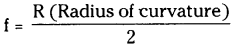Question 15.
Can we get a magnified image by a convex mirror?
No, convex mirror cannot form magnified image.

9th Class Physical Science Textbook Page No. 119

Question 16.
How can one see an image formed on the object itself?
When an object placed at centre of curvature the image should form at C in inverted position. So the image formed on the object itself.

Question 17.
Do you get an image when object is placed at F? Draw a ray diagram. Do the experiment.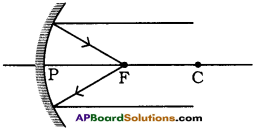When an object placed at F, we can get the image at an infinite distance.

9th Class Physical Science 7th Lesson Reflection of Light at Curved Surfaces Activities

Activity – 1

1. Observation the light rays on concave and convex surfaces.
Procedure :

1. Take a rectangular shaped (3″ x 6″) Acrylic sheet.
2. Hold the Acrylic sheet with your palm without bending it.
3. Ask your friend to focus light with a torch light on the sheet.
4. Now adjust the sheet as reflected rays are caught on a wall.
5. Now bend slowly your palm as the sheet bends inwards in the shape of concave.
7. Now bend slowly your palm as the sheet bends outwards in the shape of convex.

Observation :

1. In the first case, when without bending the sheet, reflected light is not converged at one place. Because the sheet was acted as a plane mirror.
2. In the second case, reflected light converged at one place. Because the acrylic sheet is bent inwards and it is acted as a concave mirror.
3. In the third case, reflected light rays are not converged, of course they diverged with low intensity. Because the sheet was acted as a convex mirror.

Conclusion :
Concave surfaces converge light rays and convex surfaces diverge.

Activity – 2

Question 2.
Show that a powerful source far away is needed to get parallel rays of light.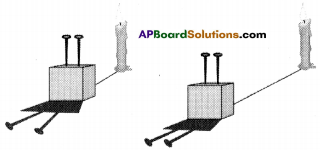1. Stick two pins on a thermocole block.
2. The pins are exactly parallel to each other.
3. When a source of light is kept very near, we see the shadows diverging (from the base of the pins).
4. As we move the source away from the pins, the angle of divergence gets reduced.
5. If we move the source far away we will get parallel shadows. But the light intensity reduces.
6. That means to get a beam of parallel rays all that we need is a powerful source far away.

Activity – 3

Question 3.
Identify the focus or focal point of concave mirror.
(OR)
How do you able to find the focal point of a given concave mirror? Explain it with an activity.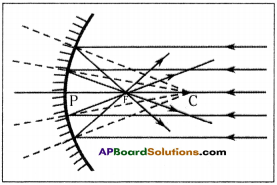1. Hold a concave mirror perpendicular to the direction of sunlight.
2. Take a small paper and slowly move it in front of the mirror and find out the point where we get smallest and brightest spot image of the sun.
3. The rays coming from the sun parallel to the concave mirror are converging at a point.
4. This point is called Focus or focal point (F) of the concave mirror.

Activity – 4

Question 4.
Find the normal to a curved surface.
(OR)
How do you find normal to a curved surface ? What is the name given to wavering point of normals, explain.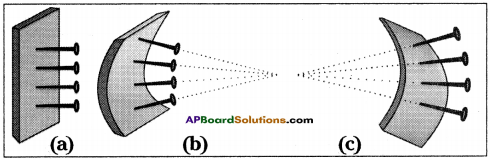• Take a small piece of thin foam or rubber (like the sole of a slipper).
• Put some pins in a straight line on the foam.
• All these pins are perpendicular to the plane of foam.
• If the foam is considered as a mirror, each pin would represent the normal at that point.
• Any ray incident at the point where the pin makes contact with the surface will reflect at the same angle as the incident ray made with the pin-normal.
• If we bend the foam piece inwards, we will notice that all the pins tend to converge at a point.
• If we bend the foam piece outwards, the pins seem to move away from each other or they diverge.
• This gives us an idea about spherical mirror.
• A concave mirror will be like the rubber sole bent inwards (fig. b) and the convex mirror will be like the rubber sole bent outwards (fig. c).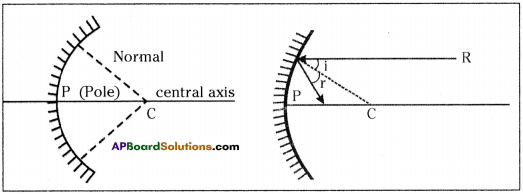• For a concave mirror, like these pins all normals will converge towards a point. This point is called centre of curvature (C) of the mirror.
• For the ray R, the incident angle is the angle it makes with the radius (normal) shown as Zi and the reflected angle is shown as Zr. We know by first law of reflection Zi = Zr.

Lab Activity

Question 5.
Write the experimented method in measure the distances of object and image using concave mirror. And write the table for observations.
(OR)
Write an experimental activity which gives the information about types of images and measuring the object distance and image distance.
Aim :
Observing the types of images formed by a concave mirror and measuring the object distance and image distance.

Material required :
A candle, paper, concave mirror, V-stand, measuring tape or meter scale.

Procedure:1) Place the concave mirror on V-stand, a candle and meter scale as shown in figure.
2) Keep the candle at different distances from the mirror (10 cm to 80 cm) along the axis and by moving the paper find the position where we get sharp image on paper.

3) Note down the observations in table -1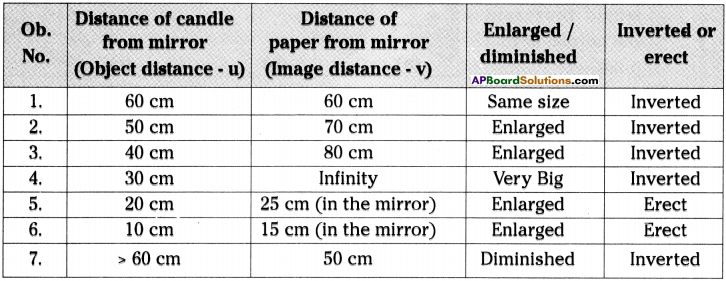4) Since we know the focal point and centre of curvature, we can re-classify the above observations as shown in the table – 2.

5) Then note down the observations in table – 2